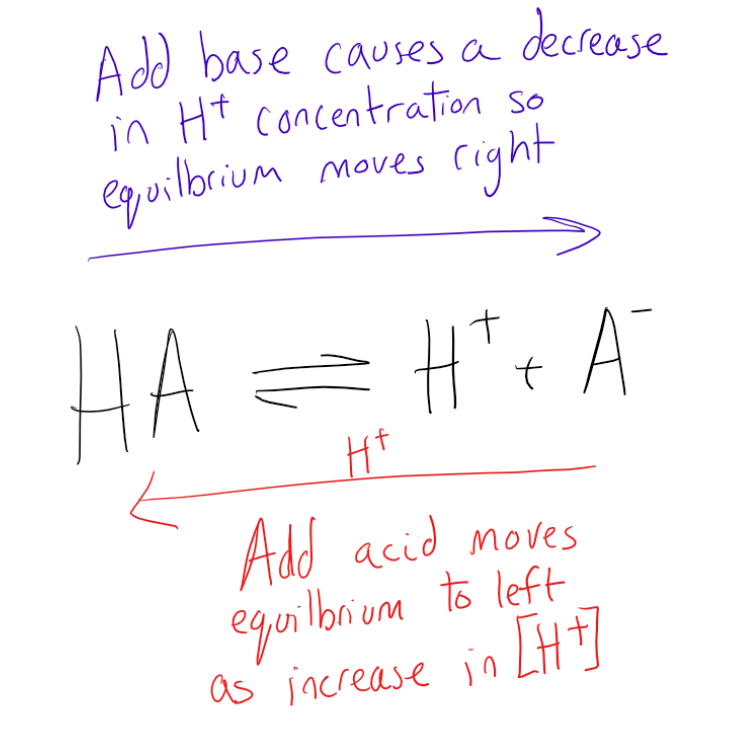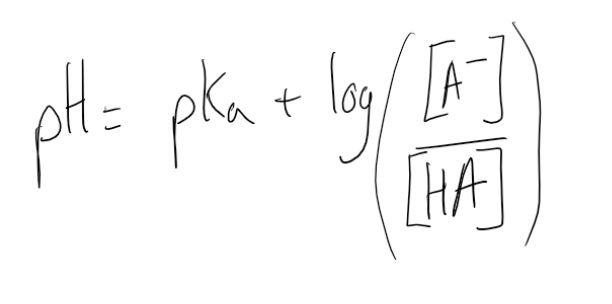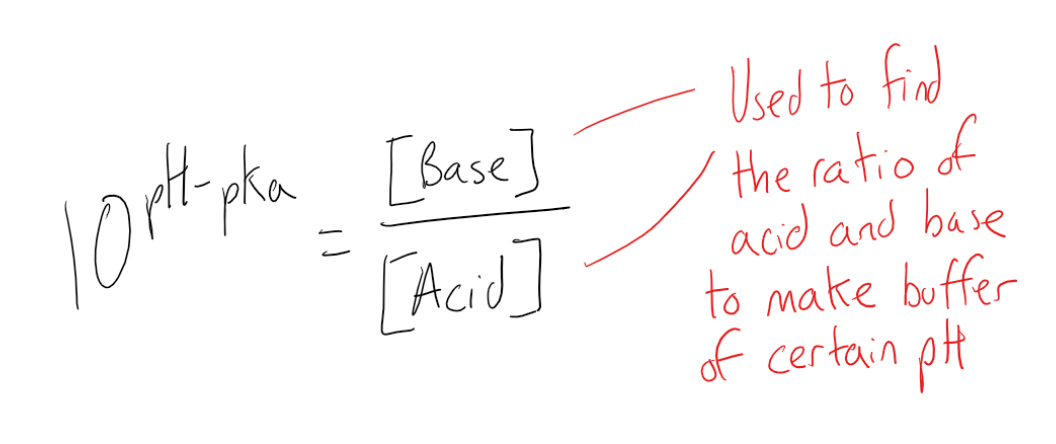Cyber Sale - Only 3 Days Remaining: ALL MCAT Products & Admissions Services

### Buffers

Topic: Acid Base Equilibria

A buffer is a solution of weak acid and conjugate base or weak base and conjugate acid used to resist pH change with the added solute.

A buffer is an aqueous solution containing a weak acid and its conjugate base or a weak base and its conjugate acid. A buffer’s pH changes very little when a small amount of strong acid or base is added to it. It is used to prevent any change in the pH of a solution, regardless of solute. Buffer solutions are used as a means of keeping pH at a nearly constant value in a wide variety of chemical applications. For example, blood in the human body is a buffer solution.

Buffer solutions are resistant to pH change because of the presence of an equilibrium between the acid (HA) and its conjugate base (A). The balanced equation for this reaction is:

HA H+ + A

When some strong acid (more H+) is added to an equilibrium mixture of the weak acid and its conjugate base, the equilibrium is shifted to the left, in accordance with Le Chatelier’s principle. This causes the hydrogen ion (H+) concentration to increase by less than the amount expected for the quantity of strong acid added. Similarly, if a strong base is added to the mixture, the hydrogen ion concentration decreases by less than the amount expected for the quantity of base added. This is because the reaction shifts to the right to accommodate for the loss of H+ in the reaction with the base.Buffer solutions are necessary in a wide range of applications. In biology, they are necessary for keeping the correct pH for proteins to work; if the pH moves outside of a narrow range, the proteins stop working and can fall apart. A buffer of carbonic acid (H2CO3) and bicarbonate (HCO3) is needed in blood plasma to maintain a pH between 7.35 and 7.45. Industrially, buffer solutions are used in fermentation processes and in setting the correct conditions for dyes used in coloring fabrics.

There are a couple of ways to prepare a buffer solution of a specific pH. In the first method, prepare a solution with an acid and its conjugate base by dissolving the acid form of the buffer in about 60% of the volume of water required to obtain the final solution volume. Then, measure the pH of the solution using a pH probe. The pH can be adjusted up to the desired value using a strong base like NaOH. If the buffer is made with a base and its conjugate acid, the pH can be adjusted using a strong acid like HCl. Once the pH is correct, dilute the solution to the final desired volume.

Alternatively, you can prepare solutions of both the acid form and base form of the solution. Both solutions must contain the same buffer concentration as the concentration of the buffer in the final solution. To get the final buffer, add one solution to the other while monitoring the pH.

Another method involves determining the exact amount of acid and conjugate base needed to make a buffer of a certain pH, using the Henderson-Hasselbach equation. The Henderson-Hasselbalch equation is useful for estimating the pH of a buffer solution and finding the equilibrium pH in an acid-base reaction:where pH is the concentration of [H+], pKa is the acid dissociation constant, and [A–] and [HA] are concentrations of the conjugate base and starting acid.

An alkaline buffer can be made from a mixture of the base and its conjugate acid, but the formulas for determining pH take a different form. The pH of bases is usually calculated using the hydroxide ion (OH) concentration to find the pOH first. The formula for pOH is pOH=-log[OH]. A base dissociation constant (Kb) indicates the strength of the base. The pH of a basic solution can be calculated by using the equation: pH = 14.00 – pOH.Buffers also affect titrations, in this case, a strong base and a weak acid or strong acid and weak base titration. The titration curve demonstrating the pH change during the titration of the strong base with a weak acid shows that at the beginning, the pH changes very slowly and gradually. This indicates the formation of a buffer system as the titration approaches the equivalence point. The initial pH of the solution at the beginning of the titration is approximately that of the weak acid in water. At the equivalence point, all of the weak acids are neutralized and converted to its conjugate base (the number of moles of H+ = added number of moles of OH–). However, the pH at the equivalence point does not equal 7. This is due to the production of the conjugate base during the titration.

Practice Questions

Qualitative analysis of contaminated water supply

MCAT Official Prep (AAMC)

Chemistry Question Pack Passage 4 Question 24

Chemistry Question Pack Passage 9 Question 47

Chemistry Question Pack Passage 9 Question 49

Sample Test C/P Section Question 58

Practice Exam 2 C/P Section Passage 8 Question 42

Practice Exam 3 C/P Section Question 26

Practice Exam 4 C/P Section Passage 8 Question 40

Key Points

• Buffer solutions are resistant to pH change because of the presence of an equilibrium between the acid (HA) and its conjugate base (A-).

• When some strong acid is added to a buffer, the equilibrium is shifted to the left, and the hydrogen ion concentration increases by less than expected for the amount of strong acid added.

• Buffer solutions are necessary in biology for keeping the correct pH for proteins to work.

• Buffers can be prepared in multiple ways by creating a solution of an acid and its conjugate base.

• A buffer is a mixture of a weak acid and its conjugate base or a base and its conjugate acid and can be used to minimize the effect of a base or acid on the pH of a solution.

• The Henderson-Hasselbalch equation is useful for estimating the pH of a buffer solution and finding the equilibrium pH in an acid-base reaction.

• The pH of bases is usually calculated using the hydroxide ion (OH) concentration to find the pOH first.

• The formula for pOH is pOH=-log[OH-]. A base dissociation constant (Kb) indicates the strength of the base.

• The pH of a basic solution can be calculated by using the equation: pH = 14.00 – pOH.

• Buffers form in titrations of strong bases and weak acids or strong acids and weak bases form buffers at the start due to the formation of the conjugate acid or base resulting in an equilibrium system establishing forming a buffer solution.

Key Terms

dissociation: the process by which compounds split into smaller constituent molecules, usually reversibly.

weak acid: one that dissociates incompletely, donating only some of its hydrogen ions into solution

weak base: a proton acceptor that does not ionize fully in an aqueous solution

aqueous: Consisting mostly of water.

equilibrium: The state of a reaction in which the rates of the forward (reactant to product) and reverse (product to reactant) reactions are the same.

pKa: A quantitative measure of the strength of an acid in solution; a weak acid has a pKa value in the approximate range −2 to 12 in water and a strong acid has a pKa value of less than about −2.

alkaline: Having a pH greater than 7.

buffers: A weak acid or base used to maintain the acidity (pH) of a solution near a chosen value and which prevent a rapid change in pH when acids or bases are added to the solution.

titration: A method or process of determining the concentration of a dissolved substance in terms of the smallest amount of reagent of known concentration required to bring about a given effect in reaction with a known volume of the test solution.

titration curve: A graph of the pH as a function of the amount of titrant (acid or base) added.

Le Chatelier’s principle: A principle stating that if a constraint (such as a change in pressure, temperature, or concentration of a reactant) is applied to a system in equilibrium, the equilibrium will shift so as to tend to counteract the effect of the constraint.

Billing Information